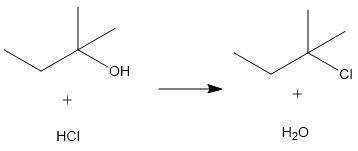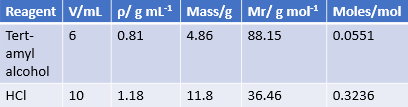# We just had a lab for nucleophilic substitution reaction and we performed a SN1 reaction for the...

## Question:

We just had a lab for nucleophilic substitution reaction and we performed a SN1 reaction for the preparation of tert amyl chloride. We used 10mL of HCl and 6mL of t-amyl alcohol. The product obtained was 1.51 grams of t-amyl chloride. The question is to calculate the percent yield and SHOW ALL WORK.

## Percentage Yields of a Reaction

The percentage yield of a reaction is the ratio of the actual yield obtained from the reaction to the theoretical yield calculated for the reaction. The equation to calculate the percentage yield is:

{eq}\%\ Yield\ =\ \frac{Actual\ Yield}{Theoretical\ Yield} \times 100\% {/eq}

It is common for the percentage yield to be less than 100%.

In order to calculate the theoretical yield, we need to:

1) Convert volumes into grams. This is done by finding the densities of the compounds and then using {eq}m=\rho \times V {/eq}, where {eq}\rho {/eq} is the density of the compound, m is its mass, and V is the volume used.

2) Calculate the moles of the substance by using the equation {eq}Moles=\frac{Mass}{Mr} {/eq}, where Mr is the molecular mass of the compound.

3) Find the limiting reagent which is the compound with the smallest number of moles used. The limiting reagent is the reagent that limits the amount of product that is formed.

4) Calculate the mass of the product that can theoretically be obtained by using the equation {eq}Mass=Mr\times Moles {/eq}

The reaction equation for this nucleophilic substitution reaction is:The table below summarises the moles of each reagent used.The limiting reagent in this reaction is, therefore, tert-amyl alcohol.

The total mass of tert-amyl chloride that can theoretically be produced is:

{eq}Mass=Mr\times Moles \\ Mass=106.59\ g\ mol^{-1} \times 0.0551\ mol \\ Mass=5.87\ g {/eq}.

Therefore, the percentage yield for this reaction is:

{eq}\%\ Yield\ =\ \frac{1.51\ g}{5.87\ g} \times 100\% \\ \%\ Yield\ =\ 25.72 \% {/eq}